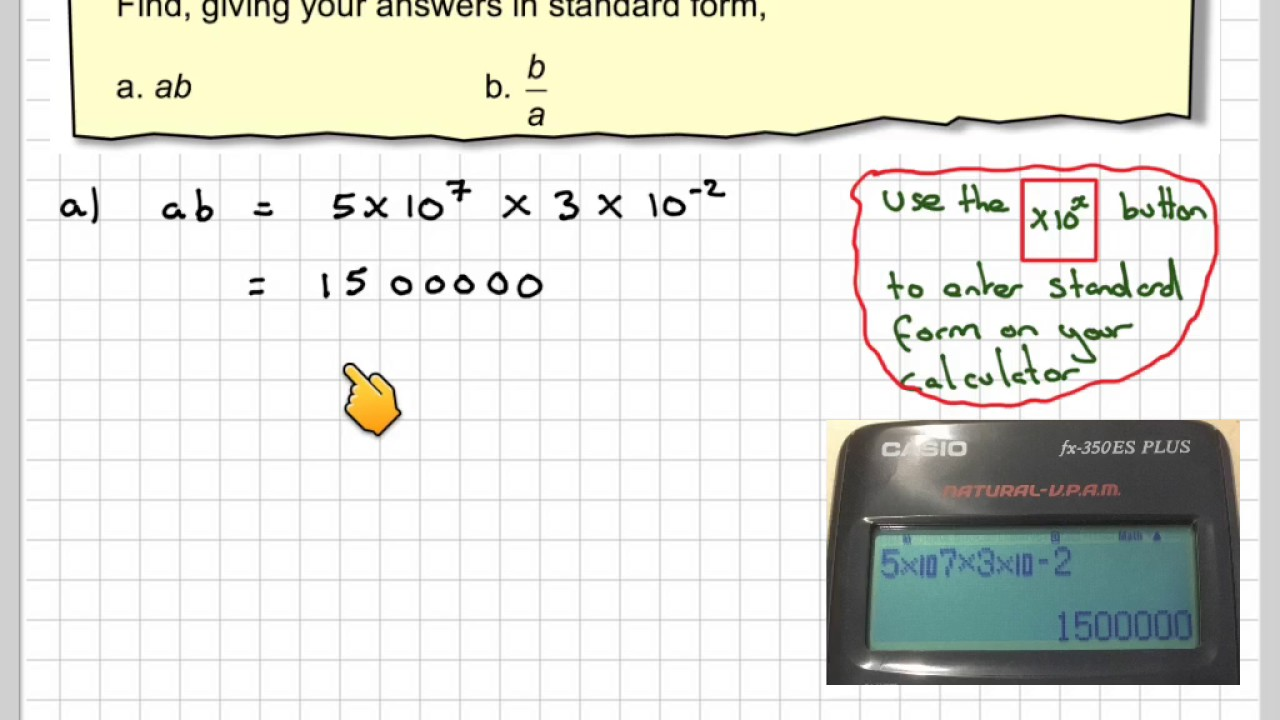# Standard Form Calculator

Standard Form Calculator is a free online tool that displays the number in the standard form. The number can be in either integer form or decimal form. BYJU’S online standard form calculator tool makes the conversion faster and easier, and it displays the standard form of the number in a fraction of seconds.

## How to Use Standard Form Calculator?

The procedure to use the standard form calculator is as follows:

Step 1: Enter the integer or decimal number in  the input field

Step 2: Now click the button “Convert” for conversion

Step 3: Finally, the standard form of the number will be displayed in the output field.

### What is the Standard Form of a Number?

The standard form of a number is introduced to avoid the difficulty of reading the large numbers. Any number that can be written in the decimal form between 1.0 to 10.0 multiplied by the power of 10. Example: 1.5× 102

### Example

Question:

Convert the number 72800000000000 into standard form.

Solution:

Given number: 72800000000000

The standard form of the given number is 7.28 × 1013